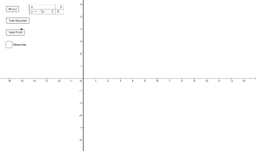# Linear equations and linear simultaneous equations

•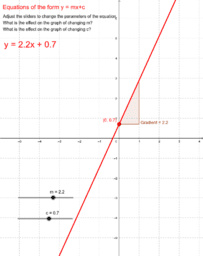### Linear equations (part 1)

•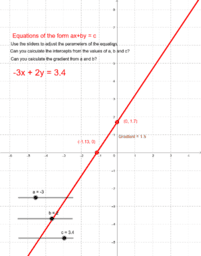### Linear equations (part 2)

•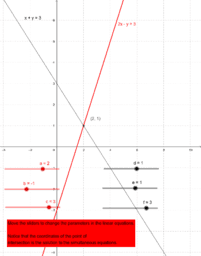### Visualisation of linear simultaneous equations

•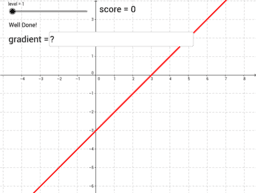•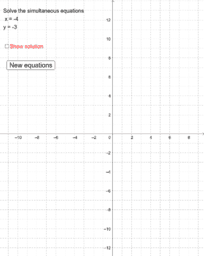### Linear simultaneous equations tester

•